# MODE Function Examples in Excel, VBA, & Google Sheets

This tutorial demonstrates how to use the Excel MODE Function in Excel to calculate the most common number.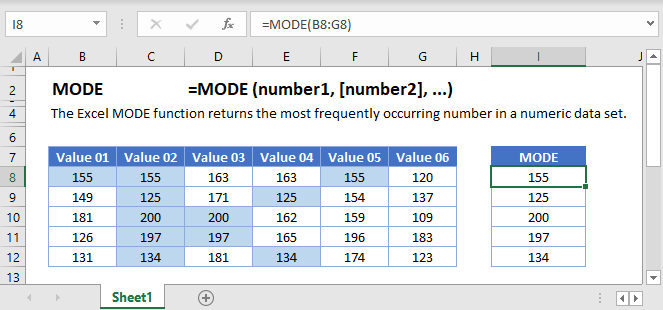MODE Function Overview
The MODE Function Calculates the most common number.

To use the MODE Excel Worksheet Function, select a cell and type: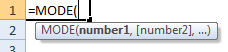(Notice how the formula inputs appear)

### MODE function Syntax and inputs:

array – An array of numbers.

## What is the mode?

The mode is the most repeated number in a group of numeric data. It also represents the central tendency of a data set, similar to mean or median.

## MODE function in Excel

The MODE function takes a range of data and returns the most frequently occurring number.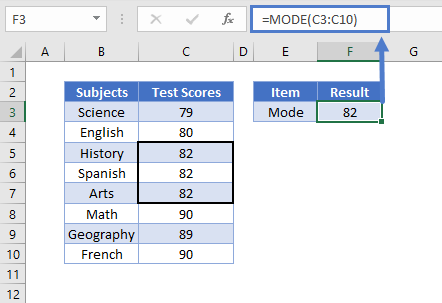If there is no mode in a group of data, the MODE function will return #N/A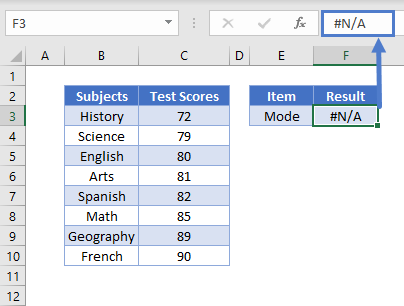## Empty Cells or Cells With Text

The MODE Function ignores cells that are empty. However, it will return #N/A error if there are non-numeric data in the group.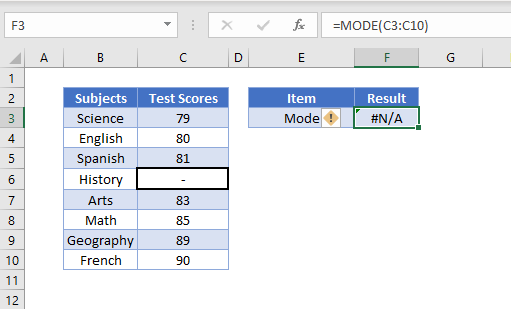Important: The MODE Function will return the same error for numbers stored as text. To use the MODE Function with numbers stored as text, first, use the VALUE Function to convert the numbers stored as text to actual numbers.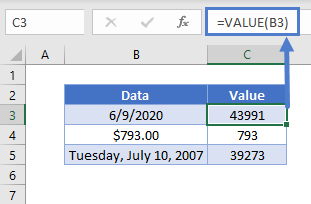## MODE.MULT and MODE.SNGL

The MODE.MULT and MODE.SNGL are new MODE Function variations available in Excel 2019.

MODE.SNGL is very similar to MODE. It is used when only one mode is present in the data set.

MODE.MULT is used when there is more than one mode present. It is an array function.

When building array functions, you must press CTRL + SHIFT + ENTER instead of just ENTER after creating your formula.

You’ll notice how the curly brackets appear. You can not just manually type in the curly brackets; you must use CTRL + SHIFT + ENTER.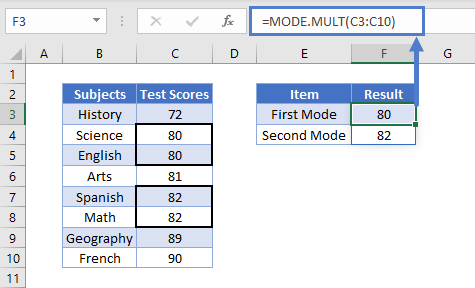Notice how the MODE.MULT function returns the result in a vertical array. We can display them in a horizontal array by using the TRANSPOSE Function. It is also an array function.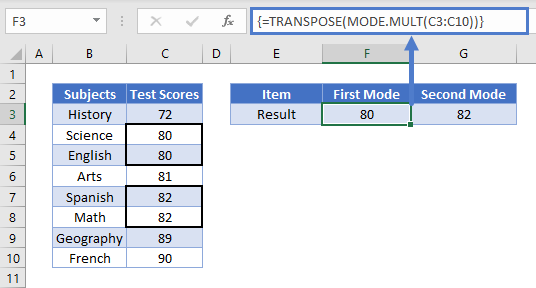## MODE function in Google Sheets

The MODE function works exactly the same in Google Sheets as in Excel.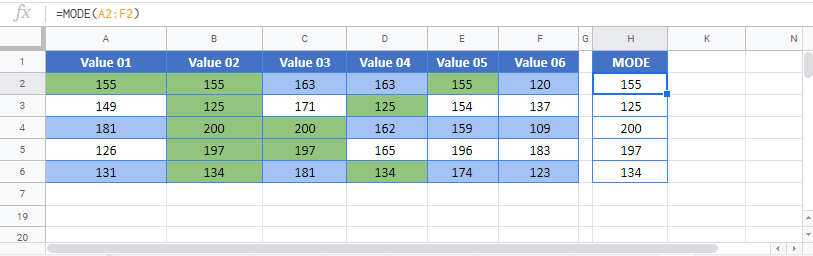## MODE Examples in VBA

You can also use the MODE function in VBA. Type:
`application.worksheetfunction.mode(number1,number2)`

Executing the following VBA statement

will produce the following results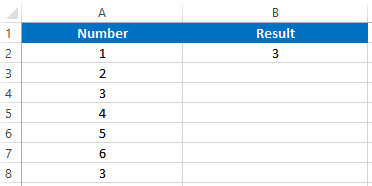For the function arguments (array, etc.), you can either enter them directly into the function, or define variables to use instead.